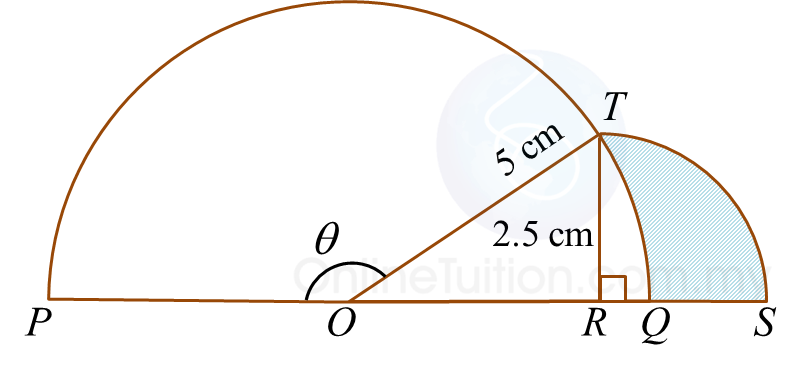# 8.5.1 Circular Measure Long Questions (Question 1 & 2)

Question 1:
Diagram shows a circle, centre O and radius 8 cm inscribed in a sector SPT of a circle at centre P.  The straight lines, SP and TP, are tangents to the circle at point Q and point R, respectively.

[Use p= 3.142]
Calculate
(a) the length, in cm, of the arc ST,
(b) the area, in cm2, of the shaded region.

Solution:
(a)

(b)

Question 2:
Diagram below shows a semicircle PTQ, with centre O and quadrant of a circle RST, with centre R.[Use π = 3.142]
Calculate
(a) the value of θ, in radians,
(b) the perimeter, in cm, of the whole diagram,
(c) the area, in cm2, of the shaded region.

Solution:

### 1 thought on “8.5.1 Circular Measure Long Questions (Question 1 & 2)”

1.this website was really useful .are there any harder questions? thanks.